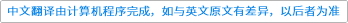International Journal of Modern Physics C ( IF 1.228 ) Pub Date : 2021-03-18 , DOI: 10.1142/s0129183121500753
T. H. Akande, F. Matthew-Ojelabi, G. S. Agunbiade, O. O. Awe

The elastic constants are paramount to determine the strength of alloys. The elastic constants of $M$–Ir, $M3$–Ir, and $M$–Ir3 where $M$ represents $Cu$, $Au$, $Ni$, $Ag$, $Pt$, $Al$, $Pd$ and $Rh$ were computed at room temperature using the embedded atom method (EAM) and the alloy mixing potentials. The potential parameters of the selected pure metals were fitted to the experimental values to compute some properties of Ni–Al, Ni3–Al, Ni–Al3, Cu–Au, Cu3–Au and Cu–Au3, and by comparing the experimental data with our predictions, the employed potential predicted some results in reasonable agreement to available experimental data with discrepancies in some cases, and these discrepancies linked to the dependence of the computed elastic constants on the fitting parameters. The potential with the metallic parameters was used as alloy parameters in computing the elastic constants, bulk modulus, and the shear modulus of the iridium binary alloy. It was generally observed that, the selected metals improve the ductility of Iridium with the highest value, recorded for Pd–Ir, and consequently the minimum value for Al–Ir, and Rh–Ir which characterized them to be ductile. The balance orders for the binary alloys were provided through the formation enthalpy.down
wechat
bug